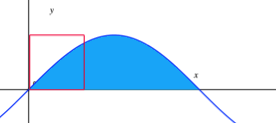# Sliding Sine Window

Calculus Level 5A square of side length one rests in the first quadrant with two of its sides sitting on the coordinate axes, overlapping the region between $y = \sin x$ and the $x$-axis. It then slides along the positive $x$-axis at a rate of 1 unit per second as shown above.

At what rate is the area inside both the blue region and the square changing after 1 second?

×